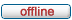# forum.alglib.net

ALGLIB forum
 It is currently Tue Feb 25, 2020 12:53 pm

 All times are UTC

### Forum rules

1. This forum can be used for discussion of both ALGLIB-related and general numerical analysis questions
2. This forum is English-only - postings in other languages will be removed.

 Print view Previous topic | Next topic
Author Message
 Post subject: CMatrixGEMM does not work proeprly???Posted: Fri Jun 19, 2015 8:08 amJoined: Fri Jun 19, 2015 8:02 am
Posts: 1
Hello,

I am using the CmatrixGemm to multiply two matrixes but it has debugging problems . I think everything is ok defined but it does not work.

Sub main()

M = 3
K = 3
N = 1
IA = 0
JA = 0
OptypeA = 0
IB = 0
JB = 0
OptypeB = 0
IC = 0
JC = 0

Dim alpha As Complex
alpha.x = 1
alpha.y = 0
Dim beta As Complex
beta.x = 0
beta.y = 0
Dim A(1 To 3, 1 To 3) As Complex
Dim B(1 To 3, 1 To 1) As Complex
Dim C(1 To 3, 1 To 1) As Complex

Dim a11 As Complex, a12 As Complex, a13 As Complex, a21 As Complex, a22 As Complex, a23 As Complex, a31 As Complex, a32 As Complex, a33 As Complex
a11.x = 1
a11.y = 0
a12.x = -0.5
a12.y = 0
a13.x = 0
a13.y = 0
a21.x = -0.5
a21.y = 0
a22.x = 1
a22.y = 0
a23.x = 0
a23.y = 0
a31.x = 0
a31.y = 0
a32.x = 0
a32.y = 0
a33.x = 0
a33.y = 3

Dim b11 As Complex, b21 As Complex, b31 As Complex
b11.x = 1
b11.y = 0
b21.x = -0.5
b21.y = 0
b31.x = 0
b31.y = 0

A(1, 1).x = a11.x: A(1, 1).y = a11.y: A(1, 2).x = a12.x: A(1, 2).y = a12.y: A(1, 3).x = a13.x: A(1, 3).y = a13.y
A(2, 1).x = a21.x: A(2, 1).y = a21.y: A(2, 2).x = a22.x: A(2, 2).y = a22.y: A(2, 3).x = a23.x: A(2, 3).y = a23.y
A(3, 1).x = a31.x: A(3, 1).y = a31.y: A(3, 2).x = a32.x: A(3, 2).y = a32.y: A(3, 3).x = a33.x: A(3, 3).y = a33.y

B(1, 1).x = b11.x: B(1, 1).y = b11.y
B(2, 1).x = b21.x: B(2, 1).y = b21.y
B(3, 1).x = b31.x: B(3, 1).y = b31.y

Call CMatrixGEMM(M, N, K, alpha, A, IA, JA, 0, B, IB, JB, 0, beta, C, IC, JC)

End Sub

TopDisplay posts from previous: All posts1 day7 days2 weeks1 month3 months6 months1 year Sort by AuthorPost timeSubject AscendingDescending

 All times are UTC

#### Who is online

Users browsing this forum: Bing [Bot] and 3 guests

 You cannot post new topics in this forumYou cannot reply to topics in this forumYou cannot edit your posts in this forumYou cannot delete your posts in this forumYou cannot post attachments in this forum

Search for: